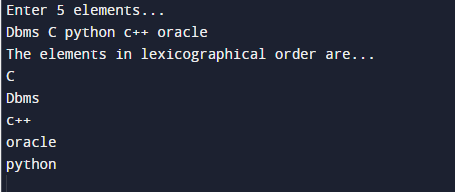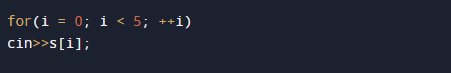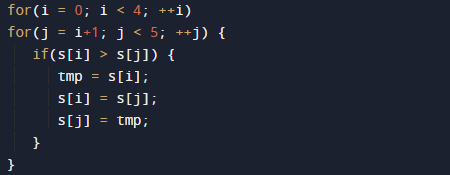C++ Program to Sort Elements in Lexicographical Order

In this tutorial you will learn about the C++ Program to Sort Elements in Lexicographical Order and its application with practical example.

In this tutorial, we will learn to create a c++ Program to Sort Elements in Lexicographical Order.

Prerequisites

Before starting with this program  we assume that you are best aware of the following C ++ programming topics:

• Operators.
• Basic input/output.
• Basic c++ programming.
• Array and Multidimensional Array.
• Loops.
• Nested For loop.

What is Lexicographical Order?.

In Maths, the terminology  lexicographic or lexicographical  order is a Alphabetical order or the Dictionary ordered,more generally “elements of a ordered set(ascending order(a-z))”.

C++ Program to Sort Elements in Lexicographical Order.

In this program we will sort elements in Lexicographical Order using nested for loop.
first of all we declared and initialized the required variables after that user will prompt to input  elements ,then we using  different methods arrange all these Elements is in Lexicographical Order.
Here we takes 5 elements from the user and arrange them in lexicographical order using nested for loop.

OutputIn the above program, we have first declared and initialized a set variables required in the program.

• s = it will holds 5 elements.
• tmp= holds temporary values.
• i and j= for iterations.

In this  program we will find Lexicographical Order of given elements by user.first of all in this statement  we will ask the user to enter the 5 elements.And we arrange the elements into Lexicographical Order using bubble sort algorithm.Bubble Sort

This sorting algorithm are also called as sinking sort method,In this sorting algorithm  terms repeatedly swapping the adjacent elements if they are in wrong order.Finally the result  printed in lexicographical order . This is given below −elements entered by user ,using algorithm we arrange them in sorted manner.In this tutorial we have learn about the C++ Program to Sort Elements in Lexicographical Order and its application with practical example. I hope you will like this tutorial.